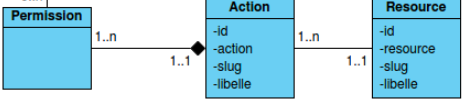We are moving our forum in GitHub Discussions. For questions about Phalcon v3/v4 you can visit here and for Phalcon v5 here.

# [UNDEFINED PROPERTY \$action] - Model relation issue

I have three models with these relations :

Permission :

``````public function initialize()
{
\$this->setSchema("ngd_demat");
\$this->setSource("p_permission");
\$this->belongsTo('idAction', Action::class, 'id', ['alias' => 'Action']);
}``````

Action :

``````public function initialize()
{
\$this->setSchema("ngd_demat");
\$this->setSource("p_action");
\$this->belongsTo('idResource', Resource::class, 'id', ['alias' => 'Resource']);
\$this->hasMany('id', Permission::class, 'idAction', ['alias' => 'Permission']);
}``````

Resource :

``````public function initialize()
{
\$this->setSchema("ngd_demat");
\$this->setSource("p_resource");
\$this->hasMany('id',Action::class,'idResource', ['alias' => 'Action']);
}``````

I want all the resource libelle :

``````public static function isAllowed(\$role){
\$resources = [];
\$permission = Permission::find('idRole ='.\$role.' AND isAllowed = 1');
foreach (\$permission->action->resource as \$resource){
array_push(\$resources, \$resource->getLibelle());
}
return \$resources;
}``````

It returns in apache error log :

Undefined property: Phalcon\Mvc\Model\Resultset\Simple::\$action

Trying to get property 'resource' of non-object

Invalid argument supplied for foreach()

This is the uml class :I've tried to put alias in lowercase and uppercase, reference model with Permission::class or "Security\Permission" or "Permission". I have Security namespace set in my loader.php.8.4k
Accepted

`Permission::find()` would return an instance of `Phalcon\Mvc\Model\Resultset`

change `Permission::find()` to `Permission::findFirst()` so it would return an instance of `Permission` model

also your `resource` relation in `Action` is a `belongsTo` so it will return an instance of `Resource` model therefore you can't loop through a single record

``````public static function isAllowed(\$role)
{
\$resources = [];

// assuming each query would return a record each time
\$permission = Permission::findFirst([
'idRole = :role: AND isAllowed = 1',
'bind' => [
'role' => \$role
]
]);

\$action = \$permission->action;

\$resource = \$action->resource;

\$resources[] = \$resource->getLibelle();

return \$resources;
}``````74

Thanks for the update and quick reply. I'll be sure to keep an eye on this thread upsers